# Plotting Pressure vs. Volume for a Thermodynamic Process

Instructor: Laura Foist

Laura has a Masters of Science in Food Science and Human Nutrition and has taught college Science.

Pressure and volume are measured in order to determine work done in some systems. In this lesson we will learn how to plot pressure and volume and what we can calculate from the plot.

## Work From Pressure and Volume

The car you drive to school is probably a gasoline engine. Gasoline engines use pistons, which use changing pressure and volume in order to get work done.

To see what is happening in a system, such as the pistons in a car, we can graph pressure vs volume, with pressure on the y-axis and volume on the x-axis. Let's look at a few different data sets. First let's see constant volume: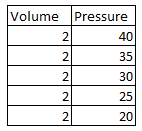When we graph this we see we get a straight vertical line: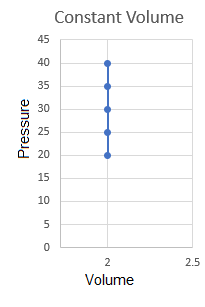Now let's look at one with constant pressure: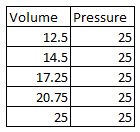When we graph this, we see we get a straight horizontal line: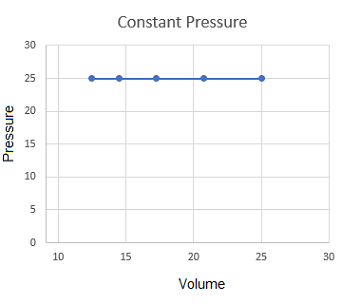Finally, let's look at one with constant temperature: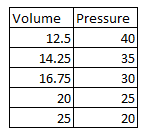When we graph this, we see we get a hyperbola: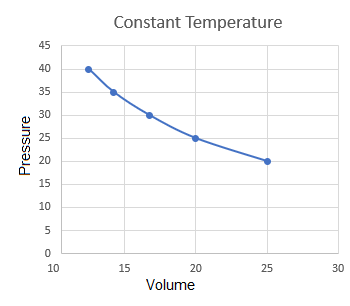These are the different general shapes we can see in a pressure vs. volume graph.

## Calculating Internal Energy

Now, let's look at the ideal gas law, where pressure times volume equals n number of moles multiplied by the universal gas constant (R) times temperature:

PV = nRT

If we are in a closed system where we aren't losing any gas particles, then n is constant, and R is always constant, so we know that PV is proportional to T. In other words as either pressure or volume is doubled, temperature is also doubled.

If both pressure and volume are doubled, then temperature is quadrupled. If pressure is doubled and volume is cut in half, then there is no change in temperature.

Now, we know that temperature is directly proportional to internal energy (U), so this means that U is also directly proportional to PV.

So, let's look at plot 1 and determine the changes in internal energy:From beginning to end the pressure is cut in half, and there are no changes in volume, so the internal energy is also cut in half. Now let's look at plot 2 and determine the changes in internal energy:From beginning to end the pressure had no changes, and the volume is doubled, so the internal energy is also doubled. Now let's look at plot 3 and determine the changes in internal energy:From beginning to end the pressure is cut in half and the temperature was doubled, which means that there were no overall changes on the internal energy, which makes sense since this is the plot for no changes in temperature.

## Calculating Work

In order to calculate work from the plot, we simply need to calculate the area under the curve. For plot 1, with constant volume, this is fairly simple:There is no area under the curve, because the change in volume is equal to 0. So no work was done. Now, let's look at plot 2:We went over 8 and up 5, so the area of the rectangle under the curve is equal to 8 x 5 = 40.

To unlock this lesson you must be a Study.com Member.

### Register to view this lesson

Are you a student or a teacher?

### Unlock Your Education

#### See for yourself why 30 million people use Study.com

##### Become a Study.com member and start learning now.
Back
What teachers are saying about Study.com

### Earning College Credit

Did you know… We have over 160 college courses that prepare you to earn credit by exam that is accepted by over 1,500 colleges and universities. You can test out of the first two years of college and save thousands off your degree. Anyone can earn credit-by-exam regardless of age or education level.